# L1-regularized quadratic function of multiple variables

## Definition

A$L^1$-regularized quadratic function of the variables$x_1,x_2,\dots,x_n$ is a function of the form (satisfying the positive definiteness condition below):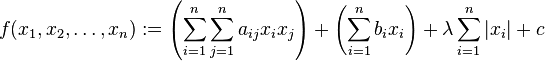$f(x_1,x_2,\dots,x_n) := \left(\sum_{i=1}^n \sum_{j=1}^n a_{ij} x_ix_j\right) + \left(\sum_{i=1}^n b_ix_i\right) + \lambda \sum_{i=1}^n |x_i| + c$

In vector form, if we denote by$\vec{x}$ the column vector with coordinates$x_1,x_2,\dots,x_n$, then we can write the function as: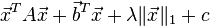$\vec{x}^TA\vec{x} + \vec{b}^T\vec{x} + \lambda\|\vec{x} \|_1 + c$

where$A$ is the$n \times n$ matrix with entries$a_{ij}$ and$\vec{b}$ is the column vector with entries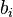$b_i$.

Note that the matrix$A$ is non-unique: if$A + A^T = F + F^T$ then we could replace$A$ by$F$. Therefore, we could choose to replace$A$ by the matrix$(A + A^T)/2$. We will thus assume that$A$ is a symmetric matrix.

We impose the further restriction that the matrix$A$ be a symmetric positive definite matrix.

## Key data

Item Value
default domain the whole of$\R^n$

## Differentiation

### Partial derivatives and gradient vector

The partial derivative with respect to the variable$x_i$, and therefore also the$i^{th}$ coordinate of the gradient vector (if it exists), is given as follows when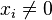$x_i \ne 0$:$\frac{\partial f}{\partial x_i} = \left(\sum_{j=1}^n (a_{ij} + a_{ji})x_j\right) + b_i + \lambda \operatorname{sgn}(x_i)$

By the symmetry assumption, this becomes: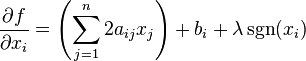$\frac{\partial f}{\partial x_i} = \left(\sum_{j=1}^n 2a_{ij}x_j\right) + b_i + \lambda \operatorname{sgn}(x_i)$

The partial derivative is undefined when$x_i = 0$.

The gradient vector exists if and only if all the coordinates are nonzero.

In vector notation, the gradient vector is as follows for all$\vec{x}$ with all coordinates nonzero:$\nabla f (\vec{x}) = 2A\vec{x} + \vec{b} + \lambda \overline{\operatorname{sgn}}(\vec{x})$

where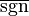$\overline{\operatorname{sgn}}$ is the signum vector function.

### Hessian matrix

The Hessian matrix of the function, defined wherever all the coordinates are nonzero, is the matrix$2A$.

## Optimization problem

We know the following two facts about the function:

• The function is a strictly convex function, and therefore it has a unique point of local minimum on the whole domain that is therefore also the point of absolute minimum. Further, it is either one of the points where the gradient vector is undefined, or it is the unique point where the gradient vector is defined and equal to zero.
• The function is a piecewise quadratic function, with the quadratic form associated with the function (namely, the quadratic term in the expression) the same in all pieces. The total number of pieces is$2^n$, namely the different orthants (the points with different sign combinations for the coordinates).

### Preliminaries

Since$A$ is a symmetric positive definite matrix, we can write$A$ in the form:$A = M^TM$

where$M$ is a$n \times n$ invertible matrix.

We can "complete the square" for this function: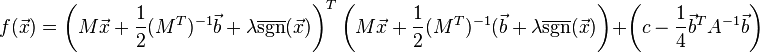$f(\vec{x}) = \left(M\vec{x} + \frac{1}{2}(M^T)^{-1}\vec{b} + \lambda \overline{\operatorname{sgn}}(\vec{x})\right)^T\left(M\vec{x} + \frac{1}{2}(M^T)^{-1}(\vec{b} + \lambda \overline{\operatorname{sgn}}(\vec{x})\right) + \left(c - \frac{1}{4}\vec{b}^TA^{-1}\vec{b}\right)$

In other words:$f(\vec{x}) = \left \| M\vec{x} + \frac{1}{2}(M^T)^{-1}\vec{b}\right \|^2 + \left(c - \frac{1}{4}\vec{b}^TA^{-1}\vec{b}\right)$

If this equals zero, that must happen at a unique point, and that point must satisfy:$M\vec{x} + \frac{1}{2}(M^T)^{-1}(\vec{b} + \lambda \overline{\operatorname{sgn}}(\vec{x})) = \vec{0}$

Simplifying, we obtain that if we can find a solution to the equation below, that is the unique point of local and absolute minimum: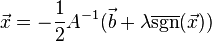$\vec{x} = -\frac{1}{2}A^{-1}(\vec{b} + \lambda \overline{\operatorname{sgn}}(\vec{x}))$

Moreover, the value of the minimum is: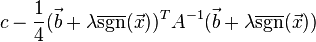$c - \frac{1}{4}(\vec{b} + \lambda \overline{\operatorname{sgn}}(\vec{x}))^TA^{-1}(\vec{b} + \lambda \overline{\operatorname{sgn}}(\vec{x}))$